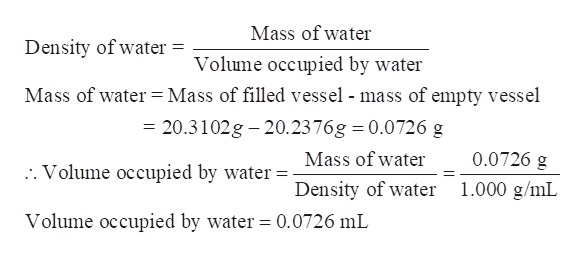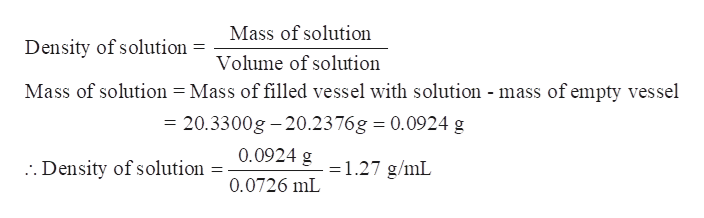A glass vessel weighed 20.2376g when empty and 20.3102g when filled to an etched mark with water at 4 degrees Celsius. The same vessel was the dried and filled to the same mark with a solution at 4 degrees Celsius. The vessel was now found to weigh 20.3300g. What is the density of the solution in g/mL? (dH20 at 4 degrees = 1.000g/mL)

Question

A glass vessel weighed 20.2376g when empty and 20.3102g when filled to an etched mark with water at 4 degrees Celsius. The same vessel was the dried and filled to the same mark with a solution at 4 degrees Celsius. The vessel was now found to weigh 20.3300g. What is the density of the solution in g/mL? (dH20 at 4 degrees = 1.000g/mL)

Step 1

A glass vessel weighed 20.2376g when empty and 20.3102g when filled to an etched mark with water at 4 degrees Celsius. The same vessel was the dried and filled to the same mark with a solution at 4 degrees Celsius. The vessel was now found to weigh 20.3300g. We have to determine the density of the solution in g/mL.

Step 2

Calculate the volume of glass vessel or water:

At 4°C,

Density of water = 1.000 g/mL

Mass of vessel with water = 20.3102 g

Mass of empty vessel = 20.2376 ghelp_outlineImage TranscriptioncloseMass of water Density of water = Volume occupied by water Mass of water = Mass of filled vessel - mass of empty vessel = 20.3102g 20.2376g 0.0726 g Mass of water 0.0726 g . Volume occupied by water Density of water 1.000 g/mL Volume occupied by water = 0.0726 mL fullscreen
Step 3

Calculate the density of given solution-

Mass of vessel with solution = 20.3300 g

Mass of empty v...help_outlineImage TranscriptioncloseMass of solution Density of solution Volume of solution Mass of solution = Mass of filled vessel with solution - mass of empty vessel = 20.3300g-20.2376g 0.0924 g . Density of solution = 0.0924 g 1.27 g/mL 0.0726 mL fullscreen

Want to see the full answer?

See Solution

Want to see this answer and more?

Our solutions are written by experts, many with advanced degrees, and available 24/7

See Solution
Tagged in

General Chemistry